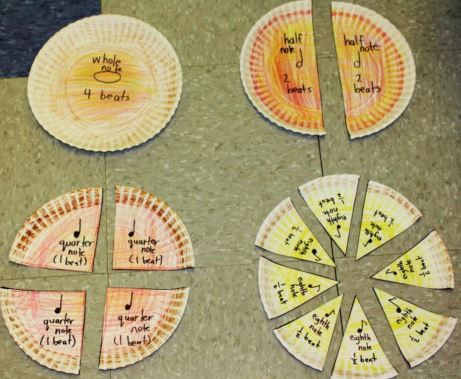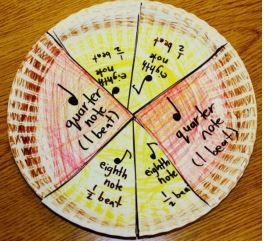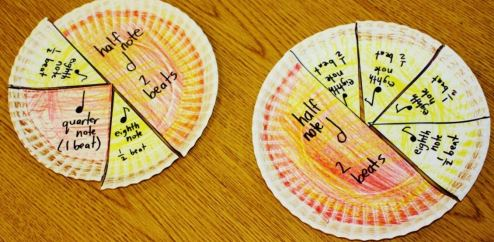Join us on Wednesday, February 3rd at 2 p.m. for a fun filled session of STEM Club where we will learn even more about music and fractions!

Before we talk about how music and fractions are related, let’s first cover some math and music vocabulary words.

### Math Vocabulary:

• Fraction – A number that shows parts of a whole or of a set.

• Denominator – The number below the line, which tells into how many parts the whole has been divided.

• Numerator – The number above the line, which indicates the number of those equal parts being considered.

• Equivalent Fraction – Fractions that have the same value or name the same amount of a whole.

• Common Denominator – A common multiple of the denominators of two or more fractions.

• Mixed Number – A number that has both a whole number part and a fractional part, such as 2 1/3. Mixed numbers represent values greater than 1.

### Music Vocabulary:

• Eighth Note/Rest – A note that lasts 1/8 the duration of a whole note. An eighth rest is silence equal to an eighth note.

• Half Note/Rest – A note that lasts 1/2 the duration of a whole note. A half rest is silence equal to a half note.

• Quarter Note/Rest– A note that lasts 1/4 the duration of a whole note. A quarter rest is silence equal to a quarter note.

• Whole Note/Rest– A musical note that lasts an entire measure in 4/4 time. A whole rest is silence equal to a whole note.

Now that we know our vocabulary words, how are fractions related to music?  As you know, fractions are parts of a whole, such as a pie or a square, or a whole anything. In music, many notes are fractions of a whole note and these notes are distinguished based on the length of time they are played. So a whole note is a length of time; a half note is half that length of time, and so on.

We’re going to work with fractions in rhythm. The most common rhythmic structure in the music we’re used to hearing is called 4-4 time. It’s also called common time because it’s…the most common! When a piece of music is in 4-4 time, it means there are four beats, or pulses, in each measure. A measure is a small section of a piece of music, just like an inch is a small section of a ruler or yardstick. Music is divided into measures, and measures are divided into beats.

We can think of one measure of music as a pizza, yes a pizza!• 1 whole note = 4 beats = 1 whole pizza
• 1 half note = 2 beats; 2 beats + 2 beats= 1 whole note (4 beats); 2 half notes = ½ pizza + ½ pizza= 1 whole pizza = 4 beats
• 1 quarter note = 1 beat; 4 quarter notes = 1 whole note (4 beats); ¼ + ¼ + ¼ + ¼ = 4/4= 1 whole pizza
• 1 eighth note = ½ beat; 2 eighth notes = 1 quarter note; 1/8 + 1/8 =2/8 = ¼;
1/8 +1/8 + 1/8 +1/8 = 2 beats = 4/8 = 1 half note = 2 quarter notes;
1/8 +1/8 + 1/8 +1/8 + 1/8 +1/8 + 1/8 +1/8 = 8/8 = 1 whole note = 1 pizza = 4 quarter notes = 2 half notes

Once you understand how each note or fraction helps add up to a whole pizza or a whole note in music you can start playing around and see how you can mix different notes/fractions to make a whole note.